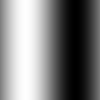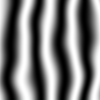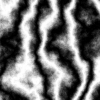## ProcGen: Sine Waves

Now that we've got a little toolkit from part 1, let's draw a simple sin wave. As you may recall from your trigonometry, the sin function goes from -1 to 1. It also loops forever. That's going to be very handy.

The `sin` function accepts an angle. Let's make the angle go from 0 to 360 as we go over the image from left to right. If you remember from last time, ix goes from 0 -> 1, but sine wants radians, 0 -> 2*pi. So let's scale the input times 2pi to make our angle theta.

``const theta = ix*2*piconst vx = sin(theta)``

Sin produces a value that goes from -1 to 1, but when we produce colors we want 0 - 1, so let's remap it as well rescale it to fit in the range we want by adding 1 (so it's 0-2) then dividing by 2. Here's the final version of rendering a sine texture

``save(map(gen(100,100), (cur,px,py,ix,iy) => {    const theta = ix*2*pi    const vx = sin(theta)    const v = (1 + vx)/2    return {r:v,g:v,b:v}}), 'v2_1.png')``Cool. Now we get an image with a single sin wave from left to right. The dark parts are the bottom of the wave and the light parts are the top. We aren't using the y value so every column has the same colors, it only varies horizontally.

### now let's have some fun.

Sin is a periodic function. It repeats. How fast it repeats is called the frequency. If we want it to repeat faster, then we need to put in an angle which changes faster. We can do that by scaling `theta` up. Let's multiply it by 4.

``save(map(gen(100,100), (cur,px,py,ix,iy) => {    const theta = ix*2*pi    const vx = sin(theta*4)    const v = (1 + vx)/2    return {r:v,g:v,b:v}}), 'v2_2.png')``

Now let's get really crazy and mix the sine waves with our noise from before. Let's add the noise to theta, essentially jittering it before calculating the sine.

``save(map(gen(100,100), (cur,px,py,ix,iy) => {    let theta = ix*2*pi    theta += octave(ix,iy,2)*1     const vx = sin(theta*4)     let v = (1 + vx)/2     return {r:v,g:v,b:v}}), 'v2_3.png')``We can scale the noise with a constant factor so that the noise has less or more effect on the sine wave. Below I increased the noise scaling so the noise has a stronger effect. I also increased the octave so the noise has greater detail.

``save(map(gen(100,100), (cur,px,py,ix,iy) => {    let theta = ix*2*pi    theta += octave(ix,iy,8)*4    const vx = sin(theta*4)    let v = (1 + vx)/2     return {r:v,g:v,b:v}}), 'v2_4.png')``That's it for today. Next time we'll color the output with gradients and lerps.